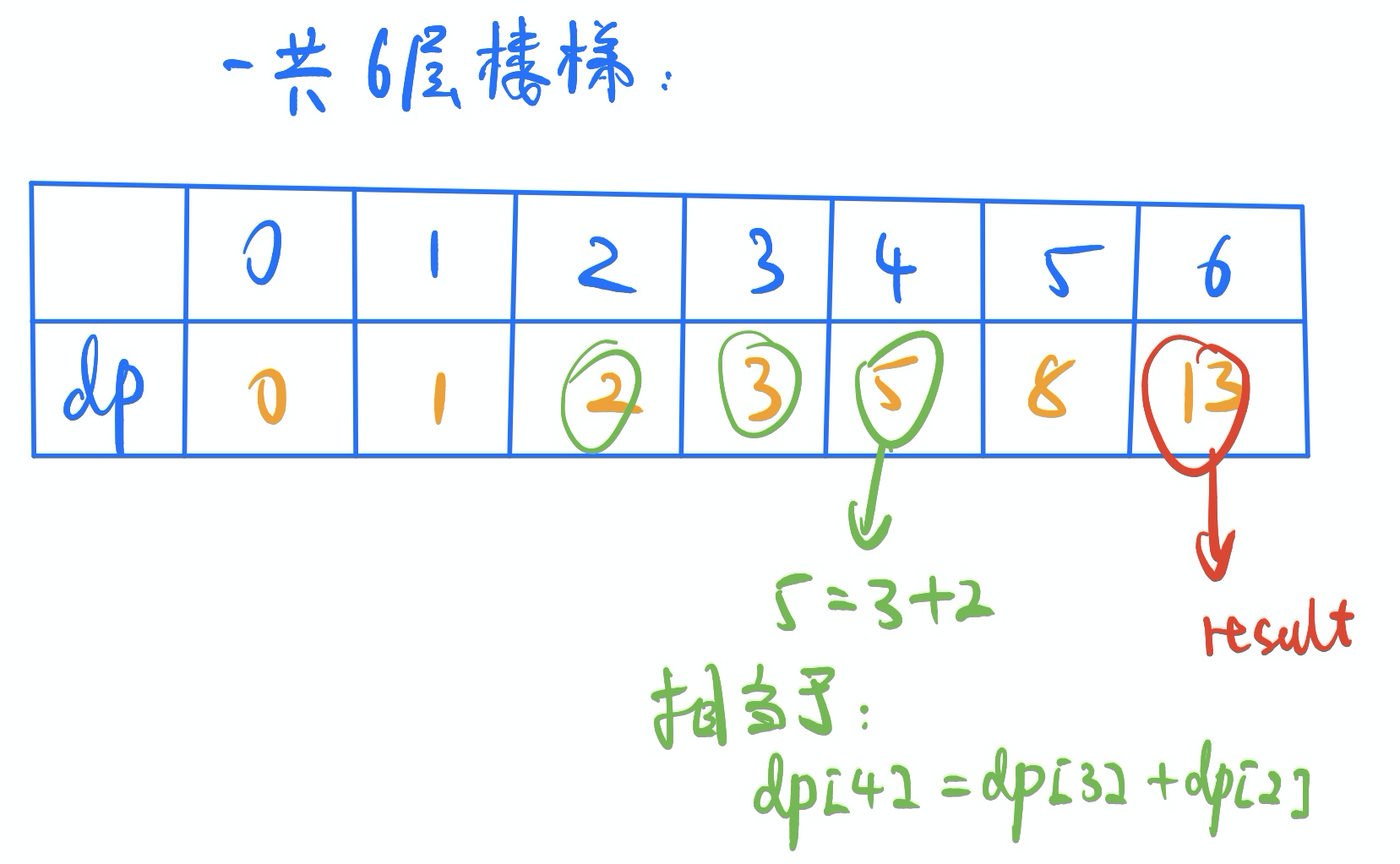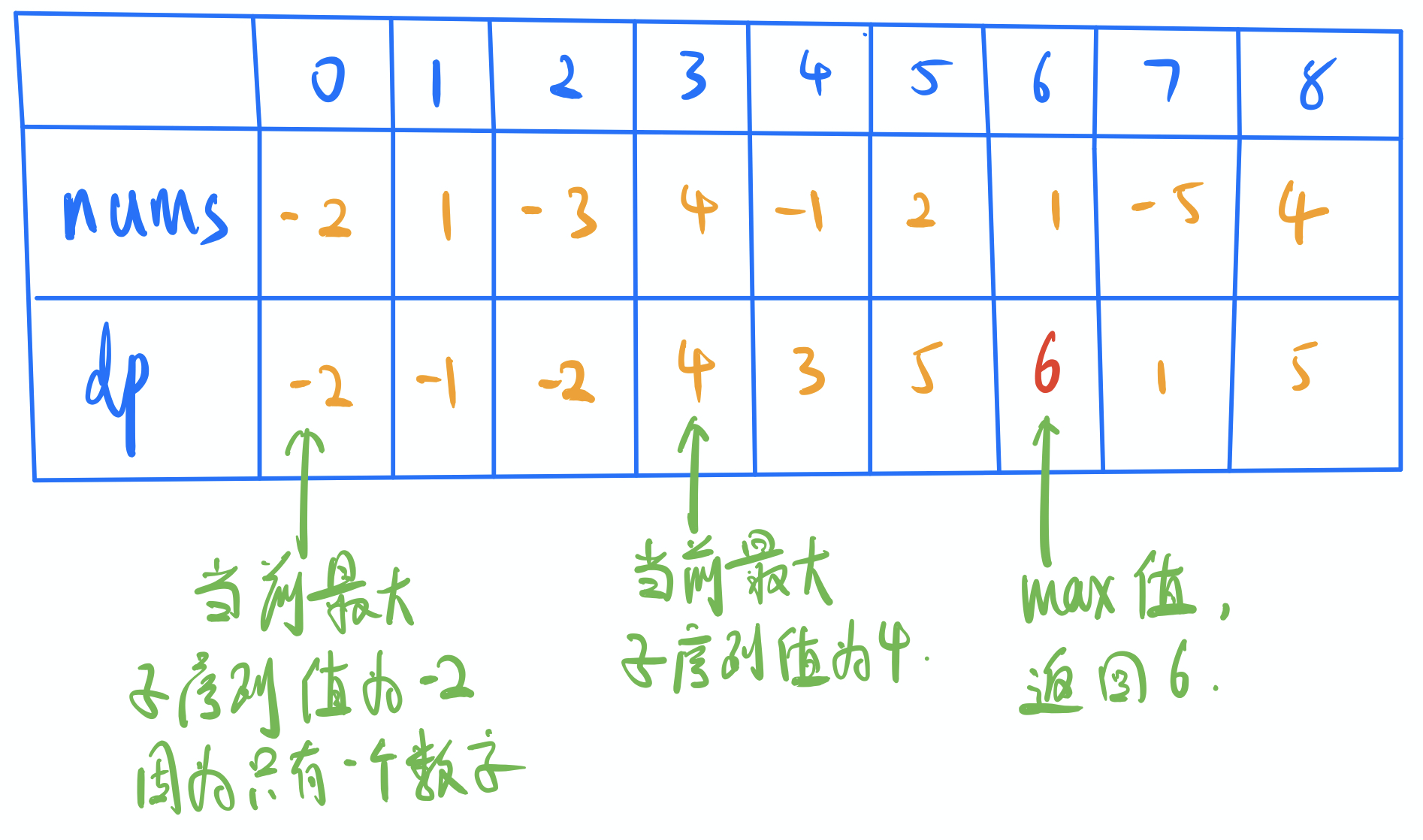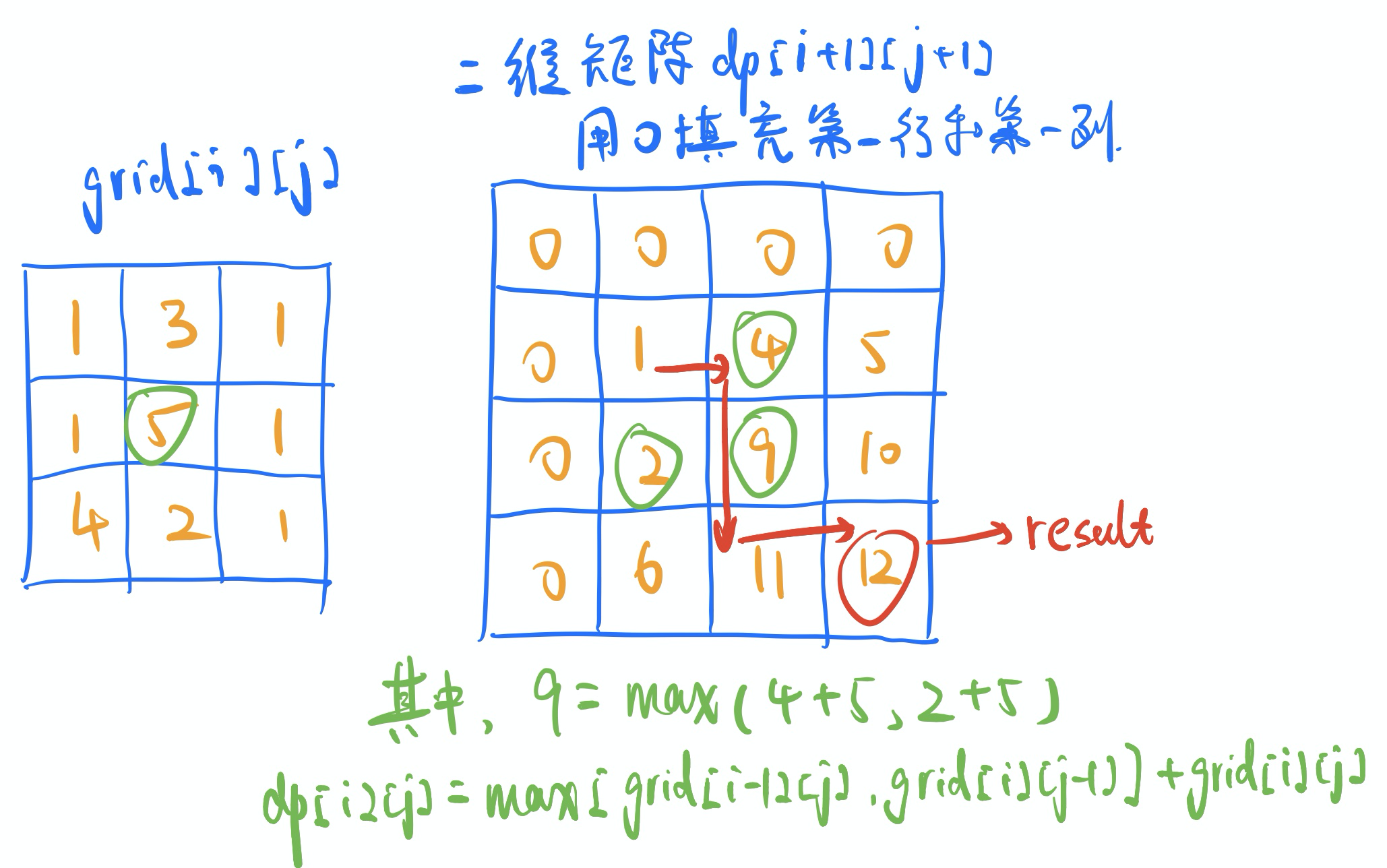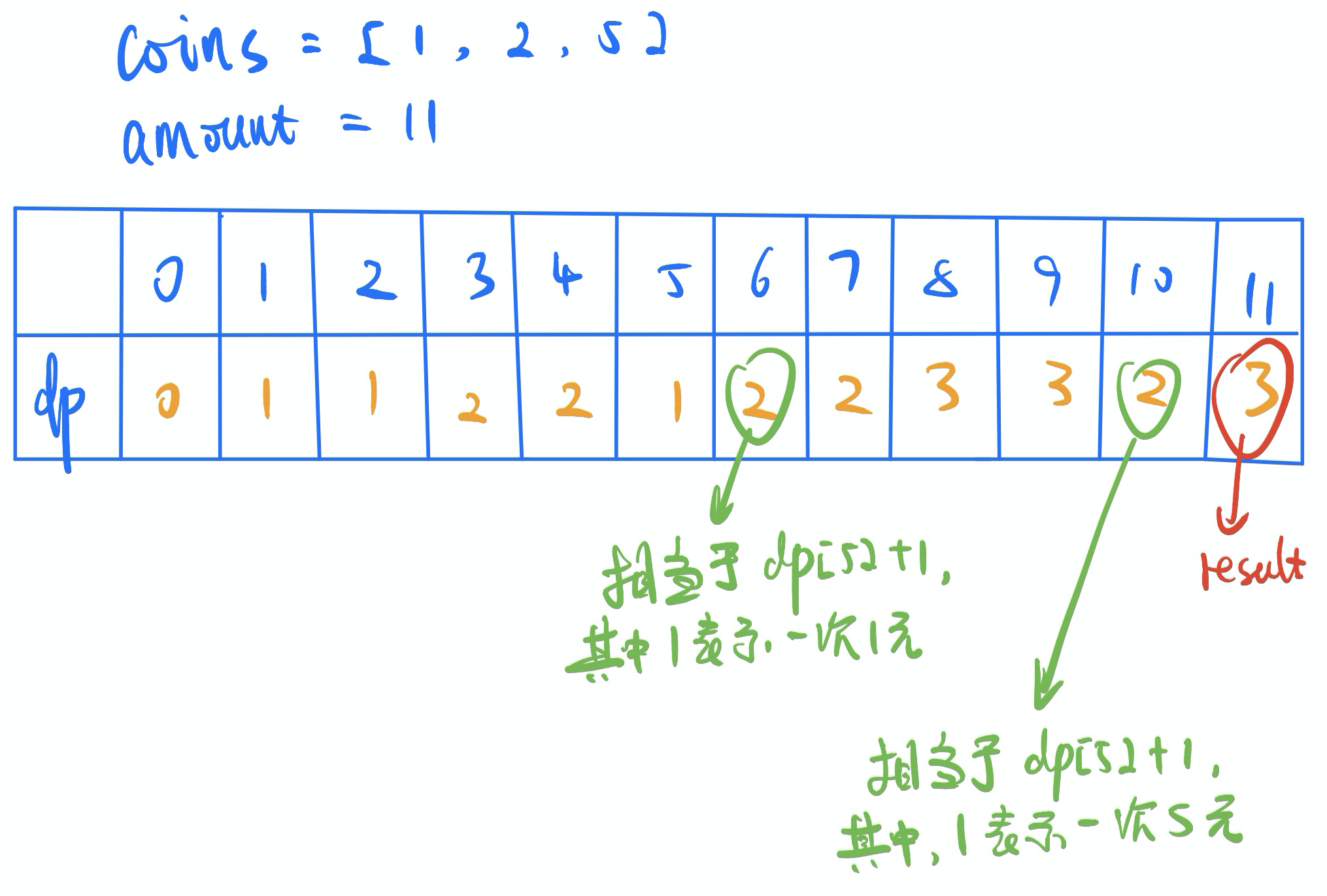• 斐波那契数列
• 一维相邻动态规划
• 二维相邻动态规划
• 间隔动态规划

一、爬楼梯

【题目链接】爬楼梯

1.  1 阶 + 1 阶
2.  2 阶【解题思路】

【代码实现】

class Solution {
public:
int climbStairs(int n) {
// 不考虑n小于2的临界值情况了
vector<int> dp(n+1);
dp = 0;
dp = 1;
dp = 2;
for (int i = 3; i <= n; i++) {
dp[i] = dp[i - 1] + dp[i - 2];
}

return dp[n];
}
};

二、连续子数组的最大和

【题目链接】连续子数组的最大和【解题思路】

dp = max(4, -2+4) = 4;
dp = max(5, 1+5) = 6;
dp[i] = std::max(dp[i-1] + nums[i], nums[i]);

【代码实现】

class Solution {
public:
int maxSubArray(vector<int>& nums) {
vector<int> dp(nums.size());
dp = nums;
int result = dp;
for (int i = 1; i < nums.size(); i++) {
dp[i] = std::max(dp[i-1] + nums[i], nums[i]);
result = std::max(dp[i], result);
}
return result;
}
};

【补充说明】

三、礼物的最大价值

【题目链接】礼物的最大价值

[
[1,3,1],
[1,5,1],
[4,2,1]
]【解题思路】

dp = max(dp, dp) + grid;
dp = max(dp, dp) + grid;
dp[i][j] = max(dp[i-1][j], dp[i][j-1]) + grid[i-1][j-1];

【代码实现】

class Solution {
public:
int maxValue(vector<vector<int>>& grid) {
int h = grid.size();    // 行数
int w = grid.size();    // 列数
if (h == 0 || w == 0) {
return 0;
}

// 生成一个二维的dp数组
vector<vector<int>> dp(h+1, vector<int>(w+1, 0));
for (int i = 0; i < h+1; i++) {
for (int j = 0; j < w+1; j++) {
if (i != 0 && j != 0) {
// 第0行和第0列值均为0，不参与计算。
dp[i][j] = max(dp[i-1][j], dp[i][j-1]) + grid[i-1][j-1];
}
}
}

return dp[h][w];
}
};

【补充说明】

【相似推荐】

四、零钱兑换

【题目链接】零钱兑换【解题思路】

【代码实现】

class Solution {
public:
int coinChange(vector<int>& coins, int amount) {
vector<int> dp(amount+1, -1);    // 所有数据，默认用-1占位，表示没有被计算过
dp = 0;
for (int i = 1; i <= amount; i++) {
for (int j = 0; j < coins.size(); j++) {
/* 如果当前面值小于i，并且i-coins[j]面额的钱也可以被拼出。
比如dp和2块钱面额，并且 dp[7-2] != -1 */
if (coins[j] <= i && dp[i-coins[j]] != -1) {
if (dp[i] == -1 || dp[i] > dp[i-coins[j]] + 1) {
dp[i] = dp[i-coins[j]] + 1;
}
}
}
}

return dp[amount];
}
};

【补充说明】

【相似推荐】

结束语

【github地址】：https://github.com/doocs/leetcode[2021.04.10 by Chunel]

个人信息

github地址: https://github.com/ChunelFeng